# SQL: Single Row Subqueries

## Single Row Subqueries

A single row subquery returns zero or one row to the outer SQL statement. You can place a subquery in a WHERE clause, a HAVING clause, or a FROM clause of a SELECT statement.

## Single Row Subqueries in WHERE clause

You can place a subquery in the WHERE clause of another query. Let's take an example of a query that contains a subquery placed in it's WHERE clause.

Sample table: agents

SQL Code:

``````SELECT agent_name, agent_code, phone_no
FROM agents
WHERE agent_code =
(SELECT agent_code
FROM agents
WHERE agent_name = 'Alex');``````

Output:

```AGENT_NAME                               AGENT_CODE PHONE_NO
---------------------------------------- ---------- ---------------
Alex                                     A003       075-12458969
```

The above example retrieves the ageent_name, agent_code, phone_no from the agents table whose agent_name is 'Alex'. Let's break the query in two parts and analyze what's going on.

SQL Code:

``````SELECT agent_code
FROM agents
WHERE agent_name = 'Alex';
``````

Relational Algebra Expression: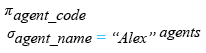Relational Algebra Tree: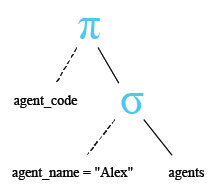Output:

```AGENT_CODE
----------
A003
```

The above subquery is executed first and returns the agent_code for the row whose agent_name is 'Alex'. The agent_code for this row is 'A003', which is passed to the where clause of the outer (main) query.
The simplified form of above code is :

SQL Code:

``````SELECT agent_name, agent_code, phone_no
FROM agents
WHERE agent_code = 'A003';``````

Relational Algebra Expression: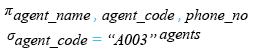Relational Algebra Tree: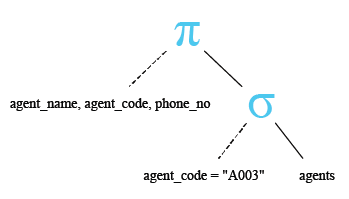Pictorical Presentation: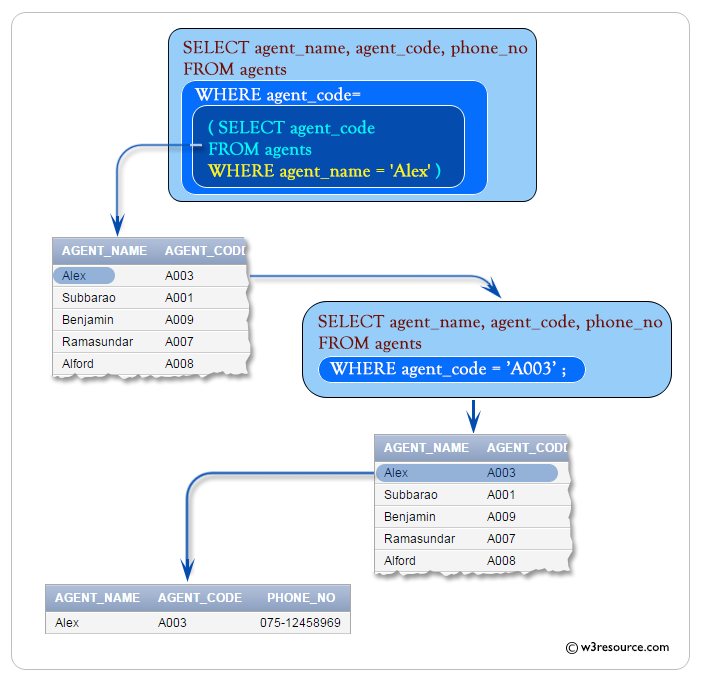## Using comparison operators in Single Row subqueries

The previous example used the equality operator (=) in the WHERE clause. Other comparison operators such as <>, >, <, <= can be used with a single subquery. The following example uses ' <' operator in the outer query WHERE clause. The AVG() function is used in the subquery to get the average order amount, which is passed to the WHERE clause of the outer query. The final result of the entire query is to get the 'ord_num', 'ord_amount', 'ord_date', 'cust_code' and 'agent_code' with following conditions:

Condition in outer query:
the 'ord_amount' of 'orders' table must be greater than the average 'ord_amount' of 'orders' table with following condition an inner join.

Condition in inner query:
'ord_date' of 'orders' table must be '20-APR-08' for calculating the average 'ord_amount'.

Sample table: orders

Here is the complete SQL statement:

SQL Code:

``````SELECT ord_num,ord_amount,ord_date,cust_code, agent_code
FROM orders
WHERE ord_amount>
(SELECT AVG(ord_amount)
FROM orders
WHERE ord_date='20-APR-08');``````

Output:

```  ORD_NUM ORD_AMOUNT ORD_DATE  CUST_CODE  AGENT_CODE
--------- ---------- --------- ---------- ----------
200114       3500 15-AUG-08 C00002     A008
200119       4000 16-SEP-08 C00007     A010
200134       4200 25-SEP-08 C00004     A005
200108       4000 15-FEB-08 C00008     A004
200109       3500 30-JUL-08 C00011     A010
200101       3000 15-JUL-08 C00001     A008
200128       3500 20-JUL-08 C00009     A002
200132       4000 15-AUG-08 C00013     A013
200110       3000 15-APR-08 C00019     A010
200107       4500 30-AUG-08 C00007     A010
200113       4000 10-JUN-08 C00022     A002
```

Let's break the query and analyze what's going on in inner query. Here is the code of inner query :

SQL Code:

``````SELECT AVG(ord_amount)
FROM orders
WHERE ord_date='20-APR-08';``````

Relational Algebra Expression: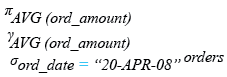Relational Algebra Tree: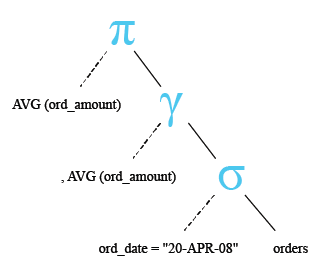Output:

```AVG(ORD_AMOUNT)
---------------
2500
```

The above query returns the average 'ord_amount' 2500, is used in the WHERE clause of the outer query shown earlier.

The simplified form of above code is:

SQL Code:

``````SELECT ord_num,ord_amount,ord_date,cust_code, agent_code
FROM orders
WHERE ord_amount>2500;``````

Relational Algebra Expression: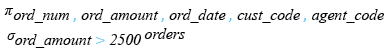Relational Algebra Tree: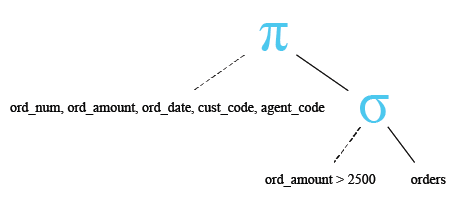Pictorical Presentation: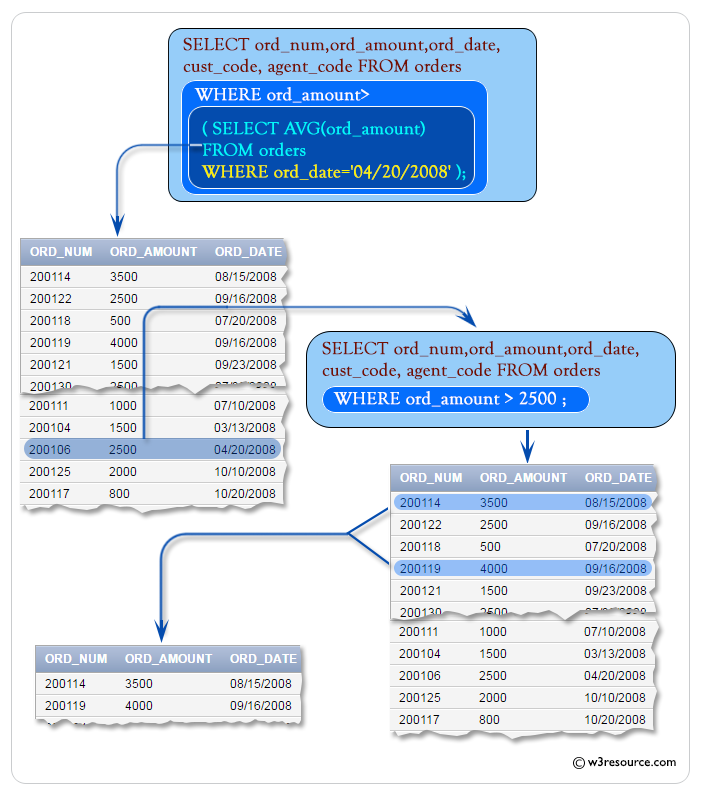## Subqueries in a HAVING clause

HAVING clause is used to filter groups of rows. You may place a subquery in HAVING clause in an outer query. This allows you to filter groups of rows based on the result returned by your subquery. The following example uses a subquery in the HAVING clause of the outer query. This example retrieves 'ord_amount, number of agent_codes and agent_code' from the table orders with following conditions:

- agent_code of orders table must come distinctly.
- an average of ord_amount of each group of agent_code in orders table must be equal to the average ord_amount of orders table.
- agent_code of orders table must be 'A008'.

Sample table: orders

Here is the complete SQL statement:

SQL Code:

``````SELECT AVG(ord_amount),COUNT(agent_code),agent_code
FROM orders
GROUP BY agent_code
HAVING AVG(ord_amount)=
(SELECT AVG(ord_amount)
FROM orders
WHERE agent_code='A008');``````

Output:

```AVG(ORD_AMOUNT) COUNT(AGENT_CODE) AGENT_CODE
--------------- ----------------- ----------
2500                 2 A011
2500                 3 A008
```

Let's break the code and analyze what's going on in inner query. Here is the code of inner query:

SQL Code:

``````SELECT AVG(ord_amount)
FROM orders
WHERE agent_code='A008';``````

Relational Algebra Expression: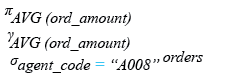Relational Algebra Tree: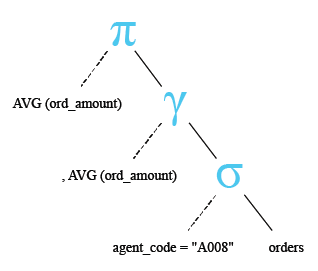Output:

```AVG(ORD_AMOUNT)
---------------
2500
```

The inner of the above query returns the average 'ord_amount' 2500.

The simplified form of the code is:

SQL Code:

``````SELECT AVG(ord_amount),COUNT(agent_code),agent_code
FROM orders
GROUP BY agent_code
HAVING AVG(ord_amount)=2500;``````

Relational Algebra Expression: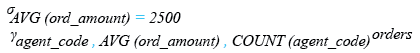Relational Algebra Tree: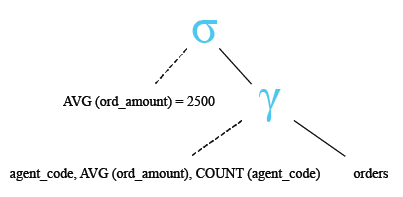Pictorial Presentation: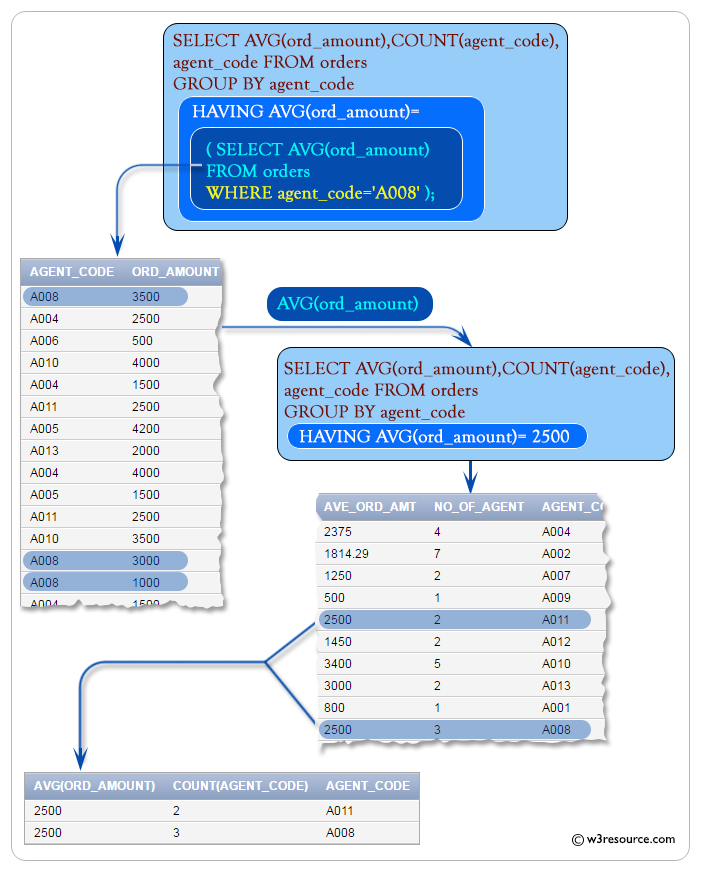## Subqueries in a FROM clause

You may place a subquery in the FROM clause of an outer query. These types of subqueries are also known is inline views because the subquery provides data inline with the FROM clause. The following example retrieves the item_id whose item_id is less than 4.

Sample table: foods

Here is the SQL statement:

SQL Code:

``````SELECT item_id
FROM
(SELECT item_id
FROM FOODS
WHERE item_id<4)``````

Output:

```ITEM_ID
------
1
2
3
```

The subquery returns the rows from the foods table whose item_id is less than 4 to the outer query, which then retrieves and display those item_id values. As far is the FROM clause of the outer query is concerned the output from the subquery is just another source of data.

Pictorical Presentation :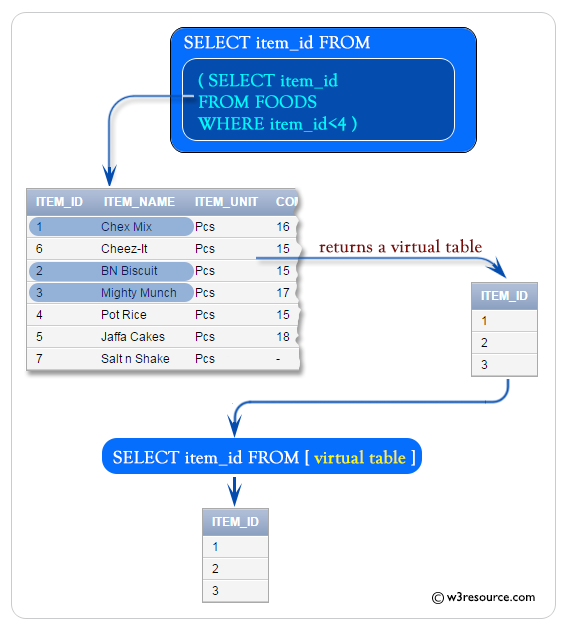## Error in Single Row Subqueries

In this section, we will discuss some errors you might face in a 'single row subquery' operation. In our previous examples, we have seen, a single row subquery always returns a single row and if a subquery returns more than one row then an error occurs. In the following example, the subquery attempts to pass multiple rows to the equality operator (=) in the outer query.

Sample table: foods

SQL Code:

``````SELECT item_id, item_name
FROM foods
WHERE item_id =
(SELECT item_id
FROM foods
WHERE item_name LIKE '%a%');``````

If you run the query in the Oracle Database 10g Express Edition you will get the following error :

ORA-01427: single-row subquery returns more than one row

Let's break the code and analyze what's going on in inner query. Here is the code of inner query:

SQL Code:

``````SELECT item_id
FROM foods
WHERE item_name LIKE '%a%';``````

Relational Algebra Expression: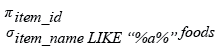Relational Algebra Tree: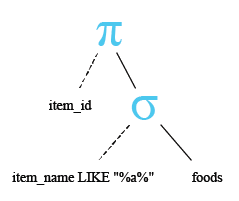Output:

```ITEM_ID
------
5
7
```

The inner query returns two rows and the subquery attempts to pass these rows to the equality operator in the outer join. Since the equality operator can handle a single row, therefore the query is invalid and an error is returned.

Pictorical Presentation: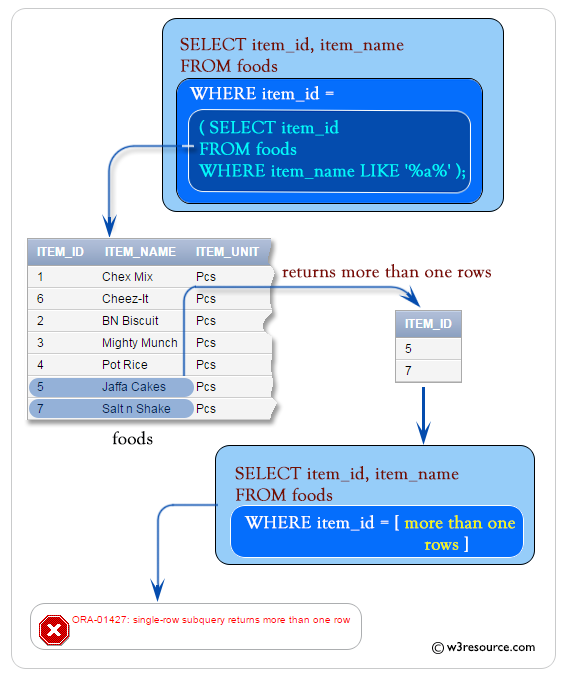See our Model Database

Practice SQL Exercises

﻿

## SQL: Tips of the Day

How to insert a line break in a SQL Server VARCHAR/NVARCHAR string?

```DECLARE @text NVARCHAR(100)
SET @text = 'This is line 1.' + CHAR(13) + 'This is line 2.'
SELECT @text
```

This prints out the following:

This is line 1.

This is line 2.

Database: SQL Server

Ref: https://bit.ly/3ry1pBI